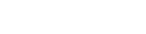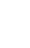# Polymorphism in JavaScript

Polymorphism is a basic feature of an object-oriented paradigm that allows you to perform a single action in several ways. It expresses the idea of being able to access objects of various kinds using the same interface. This interface can be implemented independently by each type.

``````
class A {
show() {
console.log( " A is displayed " );
}
}

class B extends A {
show() {
console.log( " B is displayed " );
}
}

class C extends A {
show() {
console.log( " C is displayed " );
}
}

var one = new A();
var two = new B();
var three = new C();

one.show();
two.show();
three.show();

``````
##### Output
``````
A is displayed
B is displayed
C is displayed

``````

## One child class dose not contain show() method

``````
class A {
show() {
console.log( " A is displayed " );
}
}

class B extends A {
show() {
console.log( " B is displayed " );
}
}

class C extends A {

}

var one = new A();
var two = new B();
var three = new C();

one.show();
two.show();
three.show();

``````
##### Output
``````
A is displayed
B is displayed
A is displayed

``````

## is-a or instanceof

A simple test may be used to determine whether an object is polymorphic. Polymorphism occurs when an item successfully passes several is-a or instanceof checks.

``````
class A {}
class B extends A {}

let val = new B();

``````
##### Output
``````
true

``````

## Object.prototype

Prototypes let you to simply specify methods for all instances of a specific object.

``````
function A() {}
let val = new A();

// changed the prototype
A.prototype = {};

``````
##### Output
``````
false

``````